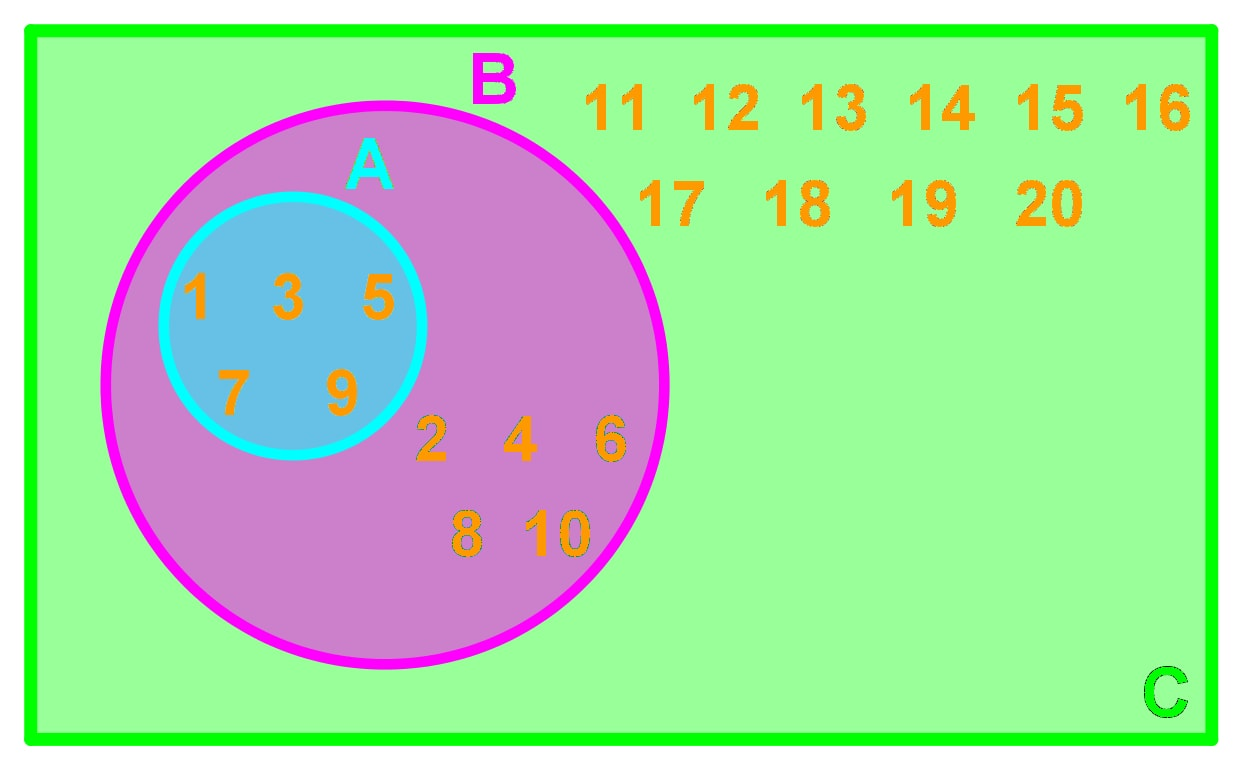# Set notation

### Set notation

#### Lessons

In this lesson, we will learn:

• Drawing Venn Diagrams With Sets
• Understanding How to Use Set Notation
• Drawing and Interpreting Venn Diagrams

Notes:

Here are some terms that we need to know for set notations:

Set: A list of objects or numbers.

Element: An object or a number in a set.

n($A$): The number of elements in set $A$.

Subset: A set where all its elements belong to another set.

Universal Set: A set of all elements in a particular context.

Empty Set: A set with no elements.

Disjoint: Two or more sets that do not have any elements in common.

Mutually Exclusive: Two or more events that cannot happen simultaneously.

Finite Set: A set with a finite number of elements.

Infinite Set: A set with an infinite number of elements.

Complement: The list of remaining elements in the universal set that is not in the mentioned set. If $B$ is a set. Then we defined the complement to be $B'$ or $\overline{B}$.

• Introduction
Set Notations Overview: Definitions and Terms

• 1.
Drawing Venn Diagrams With Sets

Consider the following information:

• $A$ = {1, 2, 3}
• $B$ = {3, 4, 5}
• Universal Set $U$ = {1, 2, 3, 4, 5, 6, 7}

Draw a Venn Diagram describing the 3 sets.

• 2.

Consider the following information:

• $A$ = {1, 2, 3}
• $B$ = {4, 5, 6}
• Universal Set $U$ = {1, 2, 3, 4, 5, 6, 7}

Draw a Venn Diagram describing the 3 sets.

• 3.
Understanding How to Use Set Notation
Consider the following information:
• Universal set $U$ = {0, 1, 2, 3, 4, 5,...}
• Set $N$ = {all natural numbers}
• Set $A$ = {0}
• Set $B$ = { }
a)
Is set $N$ a finite set or an infinite set? What about set $B$ ?

b)
List all disjoint sets, if any.

c)
Determine $n(N)$ , $n(A)$ if possible.

d)
Patsy made a statement saying that $n(A)=n(B)$ . Is this true?

e)
Is the statement $N \subset U$ true?

• 4.
Consider the following Venn Diagram:
• Universal set $U = \{\mathrm{archery, eating, chess, darts,soccer, basketball, football, volleyball, tennis, badminton}\}$
• Set $A = \{\mathrm{archery, eating, chess, darts}\}$
• Set $B = \{\mathrm{soccer, basketball, football, volleyball}\}$
a)
Explain what the sets $A,B$ and $U$ represent.

b)
List all disjoint sets, if any.

c)
List all the elements of $B'$ .

d)
Show that $n(A)+n(A')=n(U)$ .

• 5.
Consider the following Venn Diagram:a)
What is the universal set?

b)
List all the elements in set $A$ and $B$.

c)
Find a subset for set $B$.

d)
List all disjoint sets, if any.

e)
Find $n(A)$, $n(B)$, and $n(C)$.

f)
Is set $C$ a finite set?

• 6.
Drawing and Interpreting Venn Diagrams

Consider the following information:

• Universal Set $U =$ $\mathrm\{-10, -9, -8, -7, -6, -5, -4, -3, -2, -1, 0, 1, 2, 3, 4, 5, 6, 7, 8, 9, 10\}$
• Set $A$ = {positive odd number up to 10}
• Set $B$ = {positive even number up to 10}
• Set $C$ = {0}
a)
Draw a Venn diagram

b)
List all disjoint sets, if any.

c)
Find $n(A)$, $n(B)$, and $n(C)$.